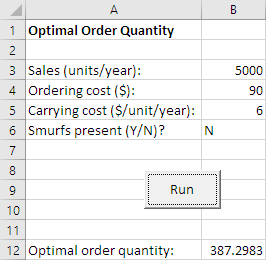# Optimal order quantity

Tags
Challenge
No

Your company buys Stuff from wholesalers, and sells it retail. Work out the optimal order quantity. That is, how many Stuffs you should order each time to minimize cost.

Here's how the worksheet starts: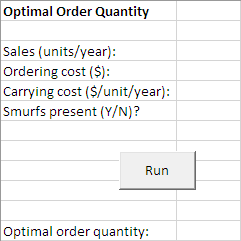Users type data into four cells. Validate them.

• Sales (units per year). Must be a number that is one or greater.
• Ordering cost (\$). The cost of placing one order. Must be a number that is not negative.
• Carrying cost (\$/unit/year). Cost of carrying a unit per year. Storage, insurance, like that. Must be a number that is greater than zero.
• Whether Smurfs are present. After trimming spaces, should be Y or N, though case doesn't matter.

Show appropriate error messages. Here's a sampling: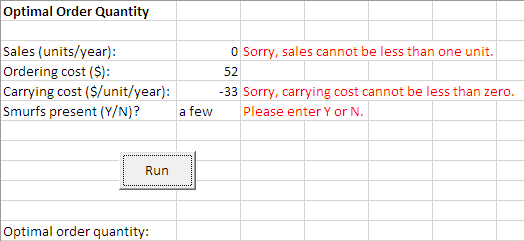All applicable error messages should show, in red, next to the cells they apply to. Use the flag pattern.

Don't show output if there are any errors. As in the screenshot above.

Here are some more errors.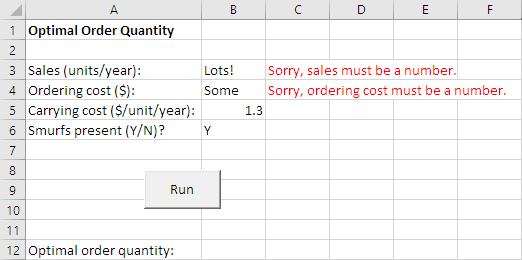If all of the data is OK, compute the optimal order quantity, and show it. The formula from Wikipedia is: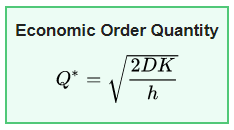Here's what the variables in the formula are: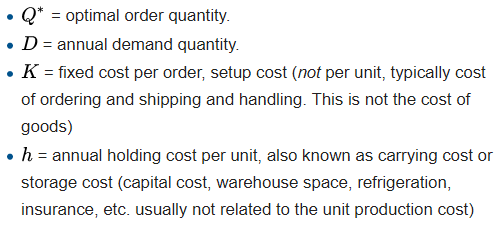If there are Smurfs, add one to the order quantity. They always steal just one.

Here's some output.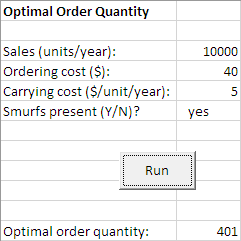Another example: